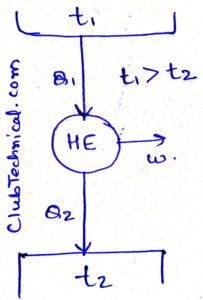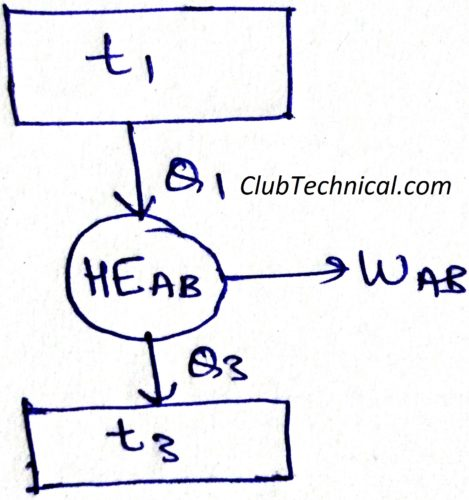# Absolute Thermodynamic Scale of Temperature

By | March 1, 2019

## Absolute thermodynamic scale of temperature

Here in this article we are going to define absolute thermodynamic scale of temperature with the help of a reversible heat engine.From the Carnot’s theorem we know that all the reversible heat engines operating between the same temperature limits have the same efficiency.

Which means that efficiency of a reversible heat engine is the function of its temperature limits.

η = f (t1, t2)   … (1)

And we also know that efficiency of any heat engine is the ratio of its work output and heat input.

η = Work output/ Heat input

η = W/Q1

η = (Q1 – Q2)/Q1

η = 1 – (Q2/Q1)   … (2)

From equation (1) and (2) we can conclude that

η = f (t1, t2) = 1 – (Q2/Q1)

We can also write above equation as

Q1/Q2 = F (t1, t2)

Note: Above relation is only valid for reversible heat engines.

Now we will use above relation in defining absolute thermodynamic scale of temperature.For the above diagram we can say that

Q1/Q2 = F (t1, t2)   … (3)

Q2/Q3 = F (t2, t3)   … (4)

Now we can replace heat engines A and B with an equivalent heat engine AB.For this case

Q1/Q3 = F (t1, t3)   … (5)

Using relation (3) and (4) in (5)

F (t1, t3) = F (t1, t2)/ F (t2, t3)   … (6)

Relation (6) is only possible if

F (t1, t3) = Ø(t1)/ Ø(t3)   … (7)

These functions Ø(t1) and Ø(t2) are defined as absolute scale of temperature.

Ø(t1)/ Ø(t3) = T1/T2

Putting this in equation (7)

F (t1, t3) = T1/T3

Putting this in equation (5)

Q1/Q3 = T1/T3

The above relation can be interpreted as

The ratio of temperature of source and sink is always equal to ratio of heat taken (from source) and heat rejected (to sink) by a reversible heat engine.